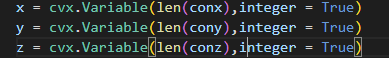# Is this a convex optimization problem? Which CVX solver be used to solve this problem?

W = 20 *10e5
F = 100 *10
conx = [5, 6]
cony = [7, 8]
conz = [9 , 6 ]
x = cvx.Variable(len(conx))
y = cvx.Variable(len(cony))
z = cvx.Variable(len(conz))
obj = cvx.Minimize(cvx.inv_pos(x) @ conx + cvx.inv_pos(y) @ cony + cvx.inv_pos(z) @ conz)
prob = cvx.Problem(obj, [x >= 0, y >= 0, cvx.sum(x) + cvx.sum(y) <= W, cvx.sum(z) <= F/10**3])
x_list = prob.solve()
print(x_list)
print(x.value)

This forum is for the MATLAB software, CVX. Please seek assistance at an appropriate CVXPY forum.

Thank you for your reply. In fact, I mainly want to ask if there is a solver to solve this optimization problem when the variable is an integer, as shown in the following figure.You should be able to use an MISOCP solver, which are available under CVXPY (and CVX).

thank you very much！